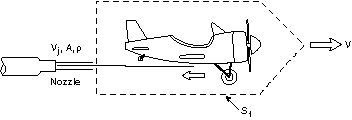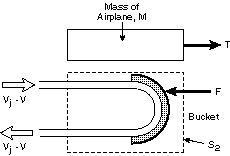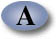Unified Engineering Spring Term 2004

Problem P2. (Propulsion) (L.O. A & B)

There is a test facility at NASA Langley Research Center where a hydraulic catapult is used to accelerate vehicles. It provides an instructive illustration of the integral momentum theorem.a) Explain what each term in the equation of motion represents.

b) Derive an equation of motion for the airplane acceleration (dV(t)/dt) as a function of the velocity, area, and density of the water jet (Vj, Aj and rj respectively), and the mass (m) and velocity (V) of the airplane. Neglect the effects of gravity and assume the forces associated with the air around the vehicle and launcher are small compared to those associated with the water jet. Assume the mass of water in contact with and accelerating the bucket is negligible relative to M so you can neglect any change with time of the momentum of the jet within the control volume.

c) What is the thrust, T, on the airplane?